# Getting Started

## Background Information

EES begins by displaying a dialog window which shows registration information, the version number and other information. The version number and registration information will be needed if you request technical support. Click the OK button to dismiss the dialog window.

Detailed help is available at any point in EES. Pressing the F1 key will bring up a Help window relating to the foremost window. Clicking the Contents button will present the Help index shown below. Clicking on an underlined word (shown in green on color monitors) will provide help relating to that subject.EES commands are distributed among nine pull-down menus. (A tenth user-defined menu can be placed to the right of the Help menu. A brief summary of their functions follows. Detailed descriptions of the commands appear in Chapter 3 of the EES manual.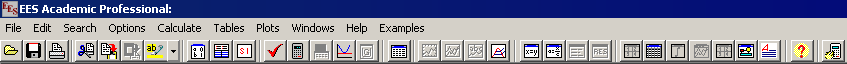Note the a toolbar is provided below the menu bar. The toolbar contains small buttons which provide rapid access to many of the most frequently used EES menu commands. If you move the cursor over a button and wait for a few second, a few words will appear to explain the function of that button. The toolbar can be hidden, if you wish, with a control in the Preferences dialog (Options menu). The System menu represented by the EES icon appears above the file menu. The System menu is not part of EES, but rather a feature of the Windows Operating System. It holds commands which allow window moving, resizing, and switching to other applications.

The Edit menu provides the editing commands to cut, copy, and paste information.

The Search menu provides Find and Replace commands for use in the Equations window.

The Options menu provides commands for setting the guess values and bounds of variables, the unit system, default information, and program preferences. A command is also provided for displaying information on built-in and user-supplied functions.

The Calculate menu contains the commands to check, format and solve the equation set.

The Tables menu contains commands to set up and alter the contents of the Parametric and Lookup Tables and to do linear regression on the data in these tables. The Parametric Table, similar to a spreadsheet, allows the equation set to be solved repeatedly while varying the values of one or more variables. The Lookup table holds user-supplied data which can be interpolated and used in the solution of the equation set.

The Plot menu provides commands to modify an existing plot or prepare a new plot of data in the Parametric, Lookup, or Array tables. Curve-fitting capability is also provided.

The Windows menu provides a convenient method of bringing any of the EES windows to the front or to organize the windows.

The basic capability provided by EES is the solution of a set of non-linear algebraic equations. To demonstrate this capability, start EES and enter this simple example problem in the Equations window. Note that EES makes no distinction between upper and lower case letters and the ^ sign (or **) is used to signify raising to a power.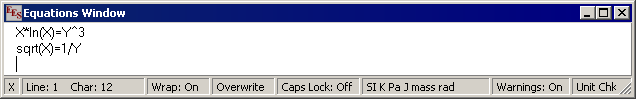If you wish, you may view the equations in mathematical notation by selecting the Formatted Equations command from the Windows menu.Select the Solve command from the Calculate menu. A dialog window will appear indicating the progress of the solution. When the calculations are completed, the button changes from Abort to Continue.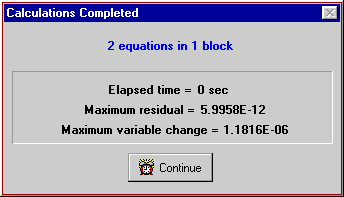Click the Continue button. The solution to this equation set will then be displayed.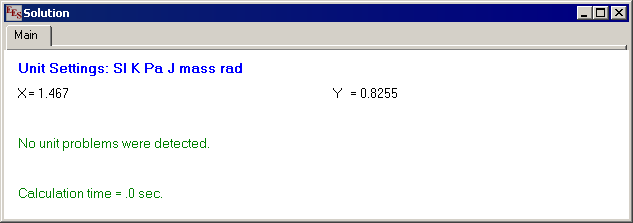## An Example Thermodynamics Problem

A simple thermodynamics problem will be set up and solved in this section to illustrate the property function access and equation solving capability of EES. The problem, typical of that which may be encountered in an undergraduate thermodynamics course, is as follows.

Refrigerant-134a enters a valve at 700 kPa, 50°C with a velocity of 15 m/s. At the exit of the valve, the pressure is 300 kPa. The inlet and outlet fluid areas are both 0.0110 m2. Determine the temperature, mass flow rate and velocity at the valve exit.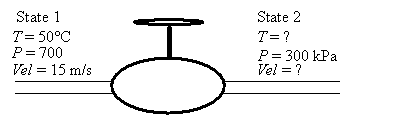To solve this problem, it is necessary to choose a system and then apply mass and energy balances. The system is the valve. The mass flow is steady, so that the mass balance is: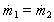where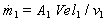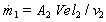m = mass flowrate [kg/s]
A = cross-sectional area [m2]
Vel = velocity [m/s]
v = specific volume [m3/kg]

We know that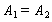The valve is assumed to be well-insulated with no moving parts. The heat and work effects are both zero. A steady-state energy balance on the valve is: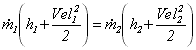where h is the specific enthalpy and Vel2/2 is the specific kinetic energy. In SI units, specific enthalpy normally has units of [kJ/kg] so some units conversions may be needed. EES provides unit conversion capabilities with the CONVERT function.

From relationships between the properties of R134a: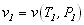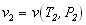Ordinarily, the terms containing velocity are neglected, primarily because the kinetic energy effects are usually small and also because these terms make the problem difficult to solve. However, with EES, the computational difficulty is not a factor. The user can solve the problem with the kinetic energy terms and judge their importance.

The values of T1, P1, A1, Vel1 and P2 are known. There are nine unknowns: A2, m1, m2, Vel2, h1, v1, h2, v2, T2. Since there are 9 equations, the solution to the problem is defined. It is now only necessary to solve the equations. This is where EES can help.

Start EES and select the New command from the File menu. A blank Equations window will appear. Before entering the equations, however, set the unit system for the built-in thermophysical properties functions. To view or change the unit system, select Unit System from the Options menu.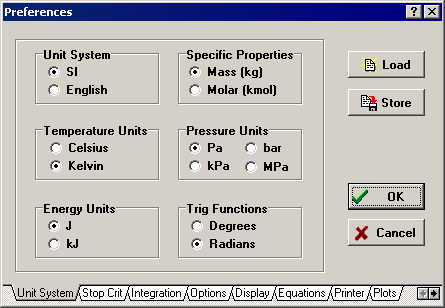EES is initially configured to be in SI units with T in °C, P in kPa, and specific property values in their customary units on a mass basis. These defaults may have been changed during a previous use. Click on the controls to set the units as shown above. Click the OK button (or press the Return key) to accept the unit system settings.

The equations can now be entered into the Equations window. Text is entered in the same manner as for any word processor. Formatting rules are as follows:

1. Upper and lower case letters are not distinguished. EES will (optionally) change the case of all variables to match the manner in which they first appear.
2. Blank lines and spaces may be entered as desired since they are ignored.
3. Comments must be enclosed within braces { } or within quote marks " ". Comments may span as many lines as needed. Comments within braces may be nested in which case only the outermost set of { } are recognized. Comments within quotes will also be displayed in the Formatted Equations window.
4. Variable names must start with a letter and consist of any keyboard characters except ( ) ' | * / + - ^ { } : " or ;. Array variables are identified with square braces around the array index or indices, e.g., X[5,3]. String variables are identified with a \$ as the last character in the variable name. The maximum length of a variable name is 30 characters.
5. Multiple equations may be entered on one line if they are separated by a semi-colon (;). The maximum line length is 255 characters.
6. The caret symbol ^ or ** is used to indicate raising to a power.
7. The order in which the equations are entered does not matter.
8. The position of knowns and unknowns in the equation does not matter.

After entering the equations for this problem and (optionally) checking the syntax using the Check/Format command in the Calculate menu, the Equations window will appear as shown. Comments are normally displayed in blue on a color monitor. Other formatting options are set with the Preferences command in the Options menu.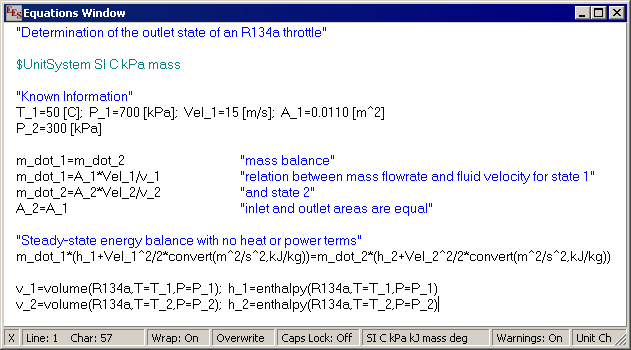EES will check the unit consistency of the equations that you enter. The units of numerical constants can be entered directly after the value. The units of variables can be entered in the Solution window, in the Variable Information dialog and in several other places. Note the use of the Convert function in this example to convert the units of the specific kinetic energy [m^2/s^2] to the units used for specific enthalpy [kJ/kg]. The Convert function is most useful in these problems.

The thermodynamic property functions, such as enthalpy and volume require a special format. The first argument of the function is the substance name, R134a in this case. The following arguments are the independent variables preceded by a single identifying letter and an equal sign. Allowable letters are T, P, H, U, S, V, and X, corresponding to temperature, pressure, specific enthalpy, specific internal energy, specific entropy, specific volume, and quality. (For psychrometric functions, additional allowable letters are W, R, D, and B, corresponding to humidity ratio, relative humidity, dewpoint temperature, and wetbulb temperature.)

An easy way to enter functions, without needing to recall the format, is to use the Function Information command in the Options menu. This command will bring up the dialog window shown below. Click on the 'Thermophysical props' radio button. The list of built-in thermophysical property function will appear on the left with the list of substances on the right. Select the property function by clicking on its name, using the scroll bar, if necessary, to bring it into view. Select a substance in the same manner. An example of the function showing the format will appear in the Example rectangle at the bottom. The information in the rectangle may be changed, if needed. Clicking the Paste button will copy the Example into the Equations window at the cursor position. Additional information is available by clicking the Info button.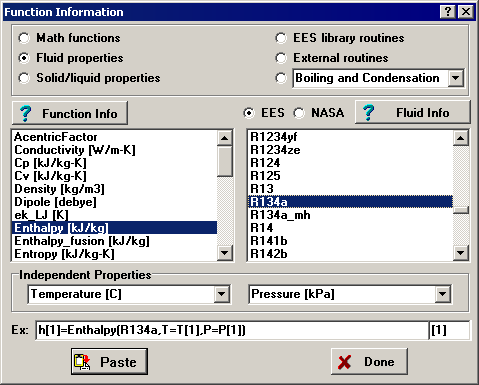It is usually a good idea to set the guess values and (possibly) the lower and upper bounds for the variables before attempting to solve the equations. This is done with the Variable Information command in the Options menu. Before displaying the Variable Information dialog, EES checks syntax and compiles newly entered and/or changed equations, and then solves all equations with one unknown. The Variable Information dialog will then appear.

The Variable Information dialog contains a line for each variable appearing in the Equations window. By default, each variable has a guess value of 1.0 with lower and upper bounds of negative and positive infinity. (If EES has previously calculated the value of the variable, the Guess value column displays the calculated value in bold font. These guess values may still be edited, which will force EES to recalculate the value of that variable.)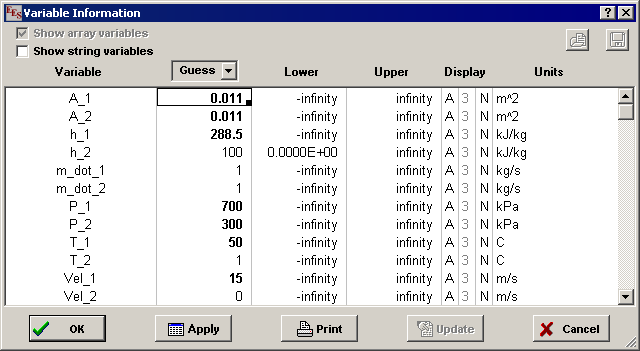The A in the Display options column indicates that EES will automatically determine the display format for numerical value of the variable when it is displayed in the Solution window. In this case, EES will select an appropriate number of digits, so the digits column to the right of the A is disabled. Automatic formatting is the default. Alternative display options are F (for fixed number of digits to the right of the decimal point) and E (for exponential format). The display and other defaults can easily be changed with the Default Information command in the Options menu, discussed in Chapter 3. The third Display options column controls the hilighting effects such as normal (default), bold, boxed. The units of the variables can be specified, if desired. The units will be displayed with the variable in the Solution window and/or in the Parametric Table. EES does not automatically do unit conversions but it can provide unit conversions using the Convert function. The units information entered here is only for display purposes.

With nonlinear equations, it is sometimes necessary to provide reasonable guess values and bounds in order to determine the desired solution. (It is not necessary for this problem.) The bounds of some variables are known from the physics of the problem. In the example problem, the enthalpy at the outlet, h_2, should be reasonably close to the value of h_1. Set its guess value to 100 and its lower bound to 0. Set the guess value of the outlet specific volume, v_2, to 0.1 and its lower bound to 0. Scroll the variable information list to bring Vel2 into view. The lower bound of Vel2 should also be zero.

To solve the equation set, select the Solve command from the Calculate menu. An information dialog will appear indicating the elapsed time, maximum residual (i.e., the difference between the left-hand side and right-hand side of an equation) and the maximum change in the values of the variables since the last iteration. When the calculations are completed, EES displays the total number of equations in the problem and the number of blocks. A block is a subset of equations which can be solved independently. EES automatically blocks the equation set, whenever possible, to improve the calculation efficiency, as described in Appendix B. When the calculations are completed, the button will change from Abort to Continue.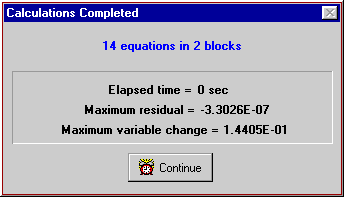By default, the calculations are stopped when 100 iterations have occurred, the elapsed time exceeds 60 sec, the maximum residual is less than 10-6 or the maximum variable change is less than 10-9. These defaults can be changed with the Stop Criteria command in the Options menu. If the maximum residual is larger than the value set for the stopping criteria, the equations were not correctly solved, possibly because the bounds on one or more variables constrained the solution. Clicking the Continue button will remove the information dialog and display the Solution window shown on the next page. The problem is now completed since the values of T_2, m_2, and Vel_2 are determined.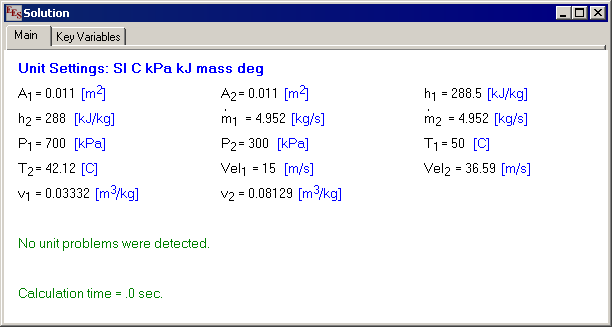One of the most useful features of EES is its ability to provide parametric studies. For example, in this problem, it may be of interest to see how the throttle outlet temperature and outlet velocity vary with outlet pressure. A series of calculations can be automated and plotted using the commands in the Tables menu.

Select the New Table command. A dialog will be displayed listing the variables appearing in the Equations window. In this case, we will construct a table containing the variables P_2, T_2, Vel_2, and h_2. Click on P_2 from the variable list on the left. This will cause P_2 to be highlighted and the Add button will become active.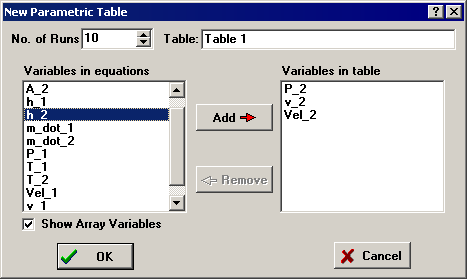Now click the Add button to move P_2 to the list of variables on the right. Repeat for T_2, h_2, and Vel_2, using the scroll bar to bring the variable into view if necessary. (As a short cut, you can double-click on the variable name in the list on the left to move it to the list on the right.). The table setup dialog should now appear as shown above. Click the OK button to create the table.

The Parametric Table works much like a spreadsheet. You can type numbers directly into the cells. Numbers which you enter are shown in black and produce the same effect as if you set the variable to that value with an equation in the Equations window. Delete the P_2 =300 [kPa] equation currently in the Equations window or enclose it in comment brackets { }. This equation will not be needed because the value of P_2 will be set in the table. Now enter the values of P_2 for which T_2 is be determined. Values of 100 to 550 have been chosen for this example. (The values could also be automatically entered using Alter Values in the Tables menu or by using the Alter Values control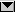at the upper right of each table column header, as explained in Chapter 2.) The Parametric Table should now appear as shown below.Now, select Solve Table from the Calculate menu. The Solve Table dialog window will appear allowing you to choose the runs for which the calculations will be done.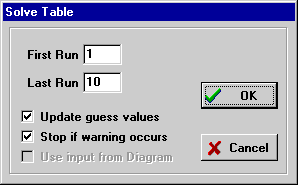When the Update Guess Values control is selected, as shown, the solution for the last run will provide guess values for the following run. Click the OK button. A status window will be displayed, indicating the progress of the solution. When the calculations are completed, the values of T_2, Vel_2, and h_2 will be entered into the table. The values calculated by EES will be displayed in blue, bold or italic type depending on the setting made in the Screen Display tab of the Preferences dialog window in the Options menu.

The relationship between variables such as P_2 and T_2 is now apparent, but it can more clearly be seen with a plot. Select New Plot Window from the Plot menu. The New Plot Window dialog window shown below will appear. Choose P_2 to be the x-axis by clicking on P_2 in the x-axis list. Click on T2 in the y-axis list. Select the scale limits for P_2 and T_2, and set the number of divisions for the scale as shown. Grid lines make the plot easier to read. Click on the Grid Lines control for both the x and y axes. When you click the OK button, the plot will be constructed and the plot window will appear as shown.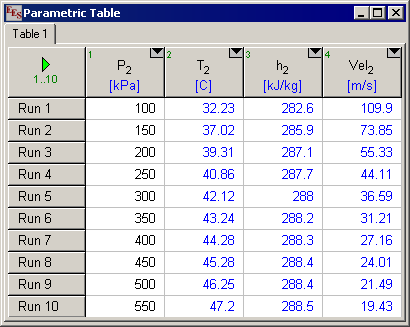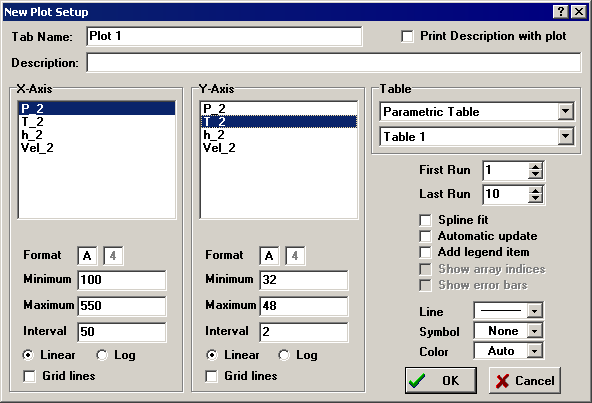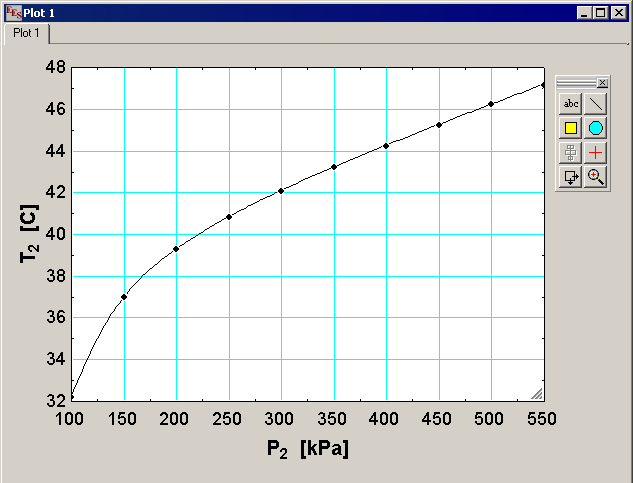Once created, there are a variety of ways in which the appearance of the plot can be changed as described in the Plot Windows section of Chapter 2 and in the Plot menu section of Chapter 3 in the EES manual.

This example problem illustrates some of the capabilities of EES. With this example behind you, you should be able to solve many types of problems. However, EES has many more capabilities and features, such as curve-fitting, uncertainty analyses, complex variables, arrays. EES will change the way you work and the way you think. If you routinely solve equations, EES is for you. EES is a general equation- solving program capable of solving hundreds of non-linear algebraic and differential equations. EES has built-in functions for the thermodynamic and transport properties of many substances and the capability for you to easily add your own functions. EES can do regression and optimization. The plotting capability in EES is often claimed to be as good or better than stand-alone plotting programs.